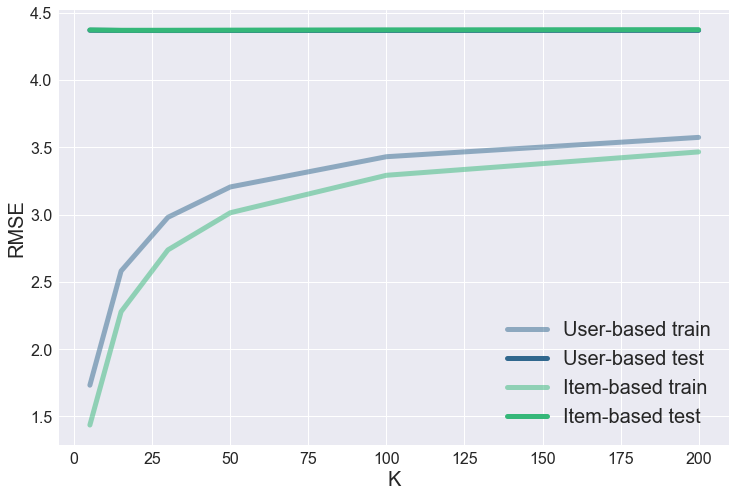Matrix-factorization,Collaborative Filtering, Singular Value Decomposition

## Introduction¶

Recommender systems are among the most popular applications of data science we see all around us. They are used to predict the "rating" or "preference" that a user would give to an item. In the retail market Amazon and ebay use it to personlize our web experience by recommending items to shoppers. Facebook uses it to recommend friends and pages to follow. Pandora uses to suggest which songs to play and Netflix recommends movies with it. A recommender system studies customer behavior like similarites between items purchased, past purchases, demographic info, their search history or movie review by consumers and use that information to predict other items to those customers. The benefits of recommender systems include improving retention,increasing sales and brand loyalty.

Among some of the most popular recommender systems include the following:

Simple recommenders: This approach generate generalized recommendations to every user based on some attributes like movie popularity and genre. The weighted average score is a common choice for building simple recommendes for movie dataset. The assumption behind this is to recommend an item to all users if based on popularity.

Content-Based Filtering The recommendation of a product is based on its discrete set of descriptive qualities.This type of recommendation system focuses on the products themselves and recommends other products that have similar attributes. For example, the site may utilize a keyword system that suggests items with similar keywords in its description to an item the user has previously purchased.T Content-based filtering relies on the characteristics of the products themselves, so it doesn’t rely on other users to interact with the products before making a recommendation.

Demographic Based Filtering This approach uses demographic information of the user such as age,gender,etc. to make recommendations.. It Prediction is done by suggessting items selected by other users that fit the same demographic pro

Utility-Based Filtering Utility Based Filtering elicits the utility that the user will get from the product. It can include information such as vendor reliability and availability to make sure it recommends products easily obtained by the user.

Knowledge-Based Filtering Recommendation selections is based on a user’s known preferences and buying patterns. Since the system will know what the consumer purchased in the past, it can make recommendations based on what might fill those needs in the future.

Hybrid Filtering This type of system combines two or more different recommender techniques to create a more thorough recommending system.

## Collaborative Filtering¶

This type of recommender system uses the preferences or ratings of other users to make predictions for other users. Collaborative filtering involves filtering for information or patterns using techniques involving collaboration among multiple agents, viewpoints, data sources, etc.Collaborative Filtering can be claasified into thre main parts, memory based CF,model based CF and hybrid recommenders.

#### Memory based approach:¶

User-Item Collaborative Filtering: The similarity between two users x and y is calculated based on similarity in patterns of items they both rated (Users like you also bought y item). An application of this user-based collaborative filtering is the Nearest Neighbor algorithm.

Item-Item Collaborative Filtering:: item-based collaborative filtering (users who bought x also bought y), proceeds in an item-centric manner:

Build an item-item matrix determining relationships between pairs of items Infer the tastes of the current user by examining the matrix and matching that user's data See, for example, the Slope One item-based collaborative filtering family.

• Item-Based Collaborative Filtering

Common measures of similarity between two users or items include the Pearson correlation and vector cosine given below:

The similarity between two users/items $x$, $y$ is measured by computing the Pearson correlation between users/items $x$, $y$ and is defined as:

\begin{equation*} \text{Similarity }(x,y)=\frac{\displaystyle \sum\limits_{i \in I_{x,y}}(r_{x,i}-\bar{r}_{x})(r_{y,i}-\bar{r}_{y})}{\sqrt{\displaystyle \sum\limits_{i \in I_{x} }(r^{2}_{x,i}-\bar{r}_{x})^{2}} \sqrt{\displaystyle \sum\limits_{i \in I_{y} }(r^{2}_{y,i}-\bar{r}_{y}})^{2}} \end{equation*}

where the $i ∈ I$ summations are over the items that both the users $x$ and $y$ have rated and $\bar{r_{x}}$ is the average rating of the co-rated items of the $x$th user.

The cosine-based approach defines the cosine-similarity (cosine of the angle between the two users/items vectors) between two users/items x and y as:

\begin{equation*} \text{Similarity }(x,y)= \text{Cosine }(\theta)=\frac{ \overrightarrow{x} \cdot \overrightarrow{y}}{||\overrightarrow {x}|| \cdot ||\overrightarrow{y}||}=\frac{\displaystyle \sum\limits_{i \in I_{x,y}}r_{x,i}r_{y,i}}{\sqrt{\displaystyle \sum\limits_{i \in I_{x} }r^{2}_{x,i}} \sqrt{\displaystyle \sum\limits_{i \in I_{y} }r^{2}_{y,i}}} \end{equation*}

For example for the vector $\overrightarrow{y}=\{a_{1},a_{2}\}$ and $\overrightarrow{x}=\{b_{1},b_{2}\}$, the vector cosine similarity $\overrightarrow{y}$ and $\overrightarrow{x}$ is geven by:

\begin{equation*} \text{Similarity }(x,y)= \text{Cosine }(\theta)=\frac{ \overrightarrow{x} \cdot \overrightarrow{y}}{||\overrightarrow {x}|| \cdot ||\overrightarrow{y}||}=\frac{a_{1}\cdot b_{1}+a_{2}\cdot b_{2}}{\sqrt{b_{1}^{2}+b_{2}^{2}} \sqrt{a_{1}^{2}+a_{2}^{2}}} \end{equation*}

Predictions can be made from the user_similarity and item_similarity matricec by applying following formula for user-based CF:

In user based approaches, the value of ratings user $u$ gives to item $i$ is calculated as an aggregation of some similar users' rating of the item:

$r_{u,i}= agg_{u^{\prime} \in U } r_{u^{\prime},i}$

where $U$ denotes the set of top $N$ users that are most similar to user $u$ who rated item $i$. Typical aggregation function includes:

\begin{equation*} \hat{r}_{u,i}= \bar{r_{u}} + \frac{\displaystyle \sum\limits_{u^{\prime} \in U }\text{Similarity }(u,u^{\prime})(r_{u^{\prime},i}-\bar{r}_{u^{\prime}})}{\displaystyle \sum\limits_{u^{\prime} \in U }\left|\text{Similarity }(u,u^{\prime})\right|} \end{equation*}

#### Model based approach :¶

In this approach, CF models are developed using machine learning algorithms to predict user’s rating of unrated items. As per my understanding, the algorithms in this approach can further be broken down into 3 sub-types.

In Model based approach, models are developed using different data mining, machine learning algorithms to predict users' rating of unrated items.

In :
%matplotlib inline
import pandas as pd
import csv
import datetime
import re
import codecs
import requests
import random as r
import matplotlib.pyplot as plt
import os, random, time, shutil, requests
import numpy as np
from skimage import transform
from matplotlib import pyplot
import glob
import numpy as np
import matplotlib.pyplot as plt
import seaborn as sns
# Hide warnings if there are any
import warnings
warnings.filterwarnings('ignore')
import seaborn as sns; sns.set()
from datetime import datetime, timedelta
from dateutil.parser import parse
from dateutil.parser import parse
from scipy import stats
from ast import literal_eval
from sklearn.feature_extraction.text import TfidfVectorizer, CountVectorizer
from sklearn.metrics.pairwise import linear_kernel, cosine_similarity
from nltk.stem.snowball import SnowballStemmer
from nltk.stem.wordnet import WordNetLemmatizer
from nltk.corpus import wordnet
from ast import literal_eval
#Surprise is a Python scikit building and analyzing recommender systems.
from surprise import Reader, Dataset, SVD, evaluate
from sklearn.metrics.pairwise import cosine_similarity
from sklearn.metrics.pairwise import linear_kernel
import warnings; warnings.simplefilter('ignore')
import missingno as msno
from sklearn.model_selection import train_test_split
from numpy import linalg as LA
import multiprocessing

In :
path='/PythonRecommenderSystem'

# Check current working directory.
retval = os.getcwd()
print ("Current working directory %s" % retval)

# Now change the directory
os.chdir( path )

# Check current working directory.
retval = os.getcwd()

print("Directory changed successfully %s" % retval)

# list files in current directory
#files = os.listdir(path)
#print(files)

Current working directory /PythonRecommenderSystem
Directory changed successfully /PythonRecommenderSystem


The pet product reviews dataset from the Amazon product dataset used in this work can be found here.This dataset contains product reviews and metadata from Amazon, including 142.8 million reviews spanning May 1996 - July 2014.

This dataset includes reviews (ratings, text, helpfulness votes), product metadata (descriptions, category information, price, brand, and image features), and links (also viewed/also bought graphs).

• reviewerID - ID of the reviewer, e.g. A2SUAM1J3GNN3B
• asin - ID of the product, e.g. 0000013714
• reviewerName - name of the reviewer
• reviewText - text of the review
• overall - rating of the product
• summary - summary of the review
• unixReviewTime - time of the review (unix time)
• reviewTime - time of the review (raw)
In :
import json

petreviews = []
for line in open('reviews_Pet_Supplies_5.json', 'r'):

In :
df=pd.DataFrame(petreviews )

Out:
asin helpful overall reviewText reviewTime reviewerID reviewerName summary unixReviewTime
0 1223000893 [0, 0] 3.0 I purchased the Trilogy with hoping my two cat... 01 12, 2011 A14CK12J7C7JRK Consumer in NorCal Nice Distraction for my cats for about 15 minutes 1294790400
1 1223000893 [0, 0] 5.0 There are usually one or more of my cats watch... 09 14, 2013 A39QHP5WLON5HV Melodee Placial Entertaining for my cats 1379116800
2 1223000893 [0, 0] 4.0 I bought the triliogy and have tested out all ... 12 19, 2012 A2CR37UY3VR7BN Michelle Ashbery Entertaining 1355875200

Equivalently, the zip file can be opened

In :
import pandas as pd
import gzip
import os

# Check current working directory.
retval = os.getcwd()
print ("Current working directory %s" % retval)

# Now change the directory
os.chdir( path )

def parse(path):
g = gzip.open(path, 'rb')
for l in g:
yield eval(l)

def getDF(path):
i = 0
df = {}
for d in parse(path):
df[i] = d
i += 1
return pd.DataFrame.from_dict(df, orient='index')

df = getDF('reviews_Pet_Supplies_5.json.gz')

Current working directory /PythonRecommenderSystem

In :
df.info()

<class 'pandas.core.frame.DataFrame'>
RangeIndex: 157836 entries, 0 to 157835
Data columns (total 9 columns):
asin              157836 non-null object
overall           157836 non-null float64
reviewText        157836 non-null object
reviewTime        157836 non-null object
reviewerID        157836 non-null object
reviewerName      156493 non-null object
summary           157836 non-null object
unixReviewTime    157836 non-null int64
dtypes: float64(1), int64(1), object(7)
memory usage: 10.8+ MB

In :
df.describe()

Out:
overall unixReviewTime
count 157836.000000 1.578360e+05
mean 4.229745 1.363741e+09
std 1.175819 3.877432e+07
min 1.000000 1.009757e+09
25% 4.000000 1.353629e+09
50% 5.000000 1.373846e+09
75% 5.000000 1.390176e+09
max 5.000000 1.406074e+09
In :
#df['reviewTime'].iloc[x][0:2]
month=[]
for i in range(df.shape):
month.append(df['reviewTime'].iloc[i][0:2])


In :
# apply a function to a list
def Month(i):
return df['reviewTime'].iloc[i][0:2]

Month=list(map(Month,range(df.shape)))

In :
# apply a function to a list
def Day(i):
return df['reviewTime'].iloc[i][3:5]

Day=list(map(Day,range(df.shape)))

In :
# apply a function to a list
def Year(i):
return df['reviewTime'].iloc[i][7:11]

Year=list(map(Year,range(df.shape)))

In :
df['Month']= pd.DataFrame(Month).astype(str).astype(int)
df['Day']= pd.DataFrame(Day)
df['Day']=df['Day'].str.replace(',', '').astype(str).astype(int)
df['Year']= pd.DataFrame(Year).astype(str).astype(int)

Out:
asin helpful overall reviewText reviewTime reviewerID reviewerName summary unixReviewTime Month Day Year
0 1223000893 [0, 0] 3.0 I purchased the Trilogy with hoping my two cat... 01 12, 2011 A14CK12J7C7JRK Consumer in NorCal Nice Distraction for my cats for about 15 minutes 1294790400 1 12 2011
1 1223000893 [0, 0] 5.0 There are usually one or more of my cats watch... 09 14, 2013 A39QHP5WLON5HV Melodee Placial Entertaining for my cats 1379116800 9 14 2013
2 1223000893 [0, 0] 4.0 I bought the triliogy and have tested out all ... 12 19, 2012 A2CR37UY3VR7BN Michelle Ashbery Entertaining 1355875200 12 19 2012
In :
df.info()

<class 'pandas.core.frame.DataFrame'>
RangeIndex: 157836 entries, 0 to 157835
Data columns (total 12 columns):
asin              157836 non-null object
overall           157836 non-null float64
reviewText        157836 non-null object
reviewTime        157836 non-null object
reviewerID        157836 non-null object
reviewerName      156493 non-null object
summary           157836 non-null object
unixReviewTime    157836 non-null int64
Month             5 non-null float64
Day               157836 non-null int64
Year              157836 non-null int64
dtypes: float64(2), int64(3), object(7)
memory usage: 14.5+ MB


Alternative if we convert the variable unixReviewTime which is in unix time format,thus the number of seconds since January 01 1970 (UTC) into a standard date format, we can easily use pandas to extract the day, month and year from the date.

In :
#
#convert unix time in seconds to date-time
df["Date_Time"] = pd.to_datetime(df["unixReviewTime"], unit='s')
df['year'] = df['Date_Time'].dt.year
df['month'] = df['Date_Time'].dt.month
df['day'] = df['Date_Time'].dt.day

Out:
asin helpful overall reviewText reviewTime reviewerID reviewerName summary unixReviewTime Date_Time year month day
0 1223000893 [0, 0] 3.0 I purchased the Trilogy with hoping my two cat... 01 12, 2011 A14CK12J7C7JRK Consumer in NorCal Nice Distraction for my cats for about 15 minutes 1294790400 2011-01-12 2011 1 12
1 1223000893 [0, 0] 5.0 There are usually one or more of my cats watch... 09 14, 2013 A39QHP5WLON5HV Melodee Placial Entertaining for my cats 1379116800 2013-09-14 2013 9 14
2 1223000893 [0, 0] 4.0 I bought the triliogy and have tested out all ... 12 19, 2012 A2CR37UY3VR7BN Michelle Ashbery Entertaining 1355875200 2012-12-19 2012 12 19

#### Check which columns have missing values¶

In :
print(df.isnull().sum())

# equivalently

#check for missing values

#print(events.apply(lambda x: sum(x.isnull()),axis=0))

print('Number of rows =  {} | Number of columns = {}'.format(df.shape, df.shape))

# percent missing
print((df.isnull().sum()/df.shape)*100)

reviewerID           0
asin                 0
reviewerName      1343
reviewText           0
overall              0
summary              0
unixReviewTime       0
reviewTime           0
dtype: int64
Number of rows =  157836 | Number of columns = 9
reviewerID        0.000000
asin              0.000000
reviewerName      0.850883
reviewText        0.000000
overall           0.000000
summary           0.000000
unixReviewTime    0.000000
reviewTime        0.000000
dtype: float64


#### Visualize Unique Review Column¶

In :
bg_color = (0.5, 0.5, 0.5)

sns.set(rc={"font.style":"normal",
"axes.facecolor":bg_color,
"axes.titlesize":30,
"figure.facecolor":bg_color,
"text.color":"black",
"xtick.color":"black",
"ytick.color":"black",
"axes.labelcolor":"black",
"axes.grid":False,
'axes.labelsize':30,
'figure.figsize':(10.0, 10.0),
'xtick.labelsize':25,
'ytick.labelsize':20})

#plt.rcParams.update(params)
sns.countplot(df["overall"],palette="Blues")
plt.xlabel('rating ')
plt.title('rating of the product ')
plt.show()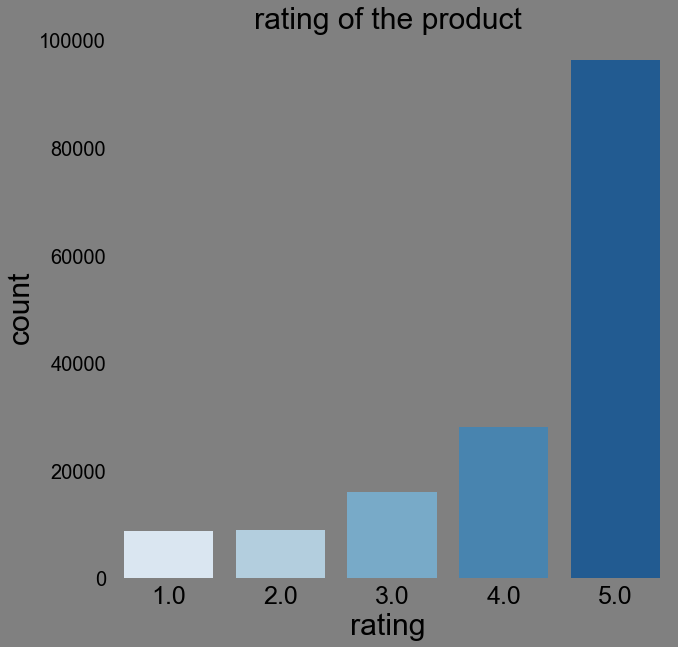#### Visualize missing Values¶

There are no missing observations in the data.

In :
msno.bar(df)
plt.show()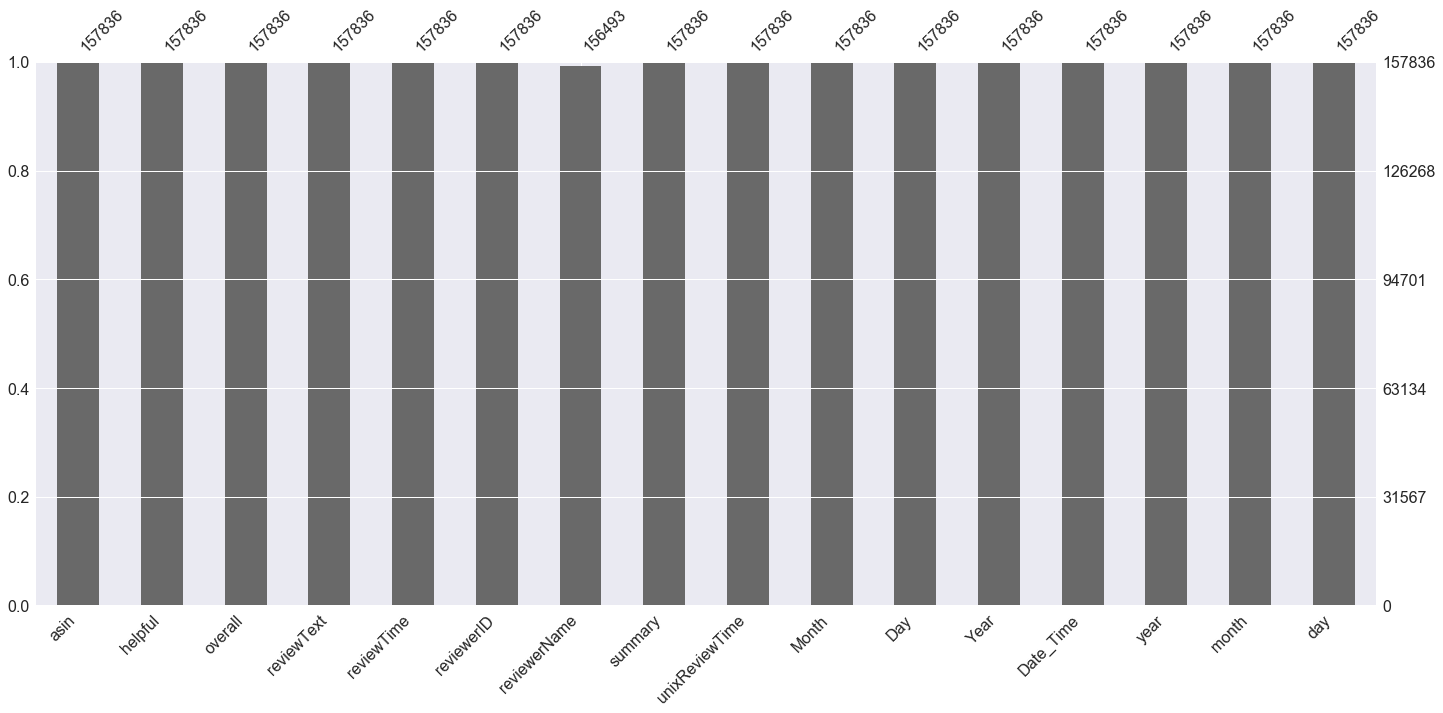#### check for correlation among variables¶

We use the seaborn heatmap function heatmap to visualize the correlations between the variables. Common choices of cmap include inferno,viridis,YlGnBu,Blues,BuPu and Greens.

In :
corr = df[df.columns].corr()

sns.set(rc={"font.style":"normal",
"axes.titlesize":30,
"text.color":"black",
"xtick.color":"black",
"ytick.color":"black",
"axes.labelcolor":"black",
"axes.grid":False,
'axes.labelsize':30,
'figure.figsize':(10.0, 10.0),
'xtick.labelsize':20,
'ytick.labelsize':20})
sns.heatmap(corr,annot = True, annot_kws={"size": 15},cmap="viridis")

plt.show()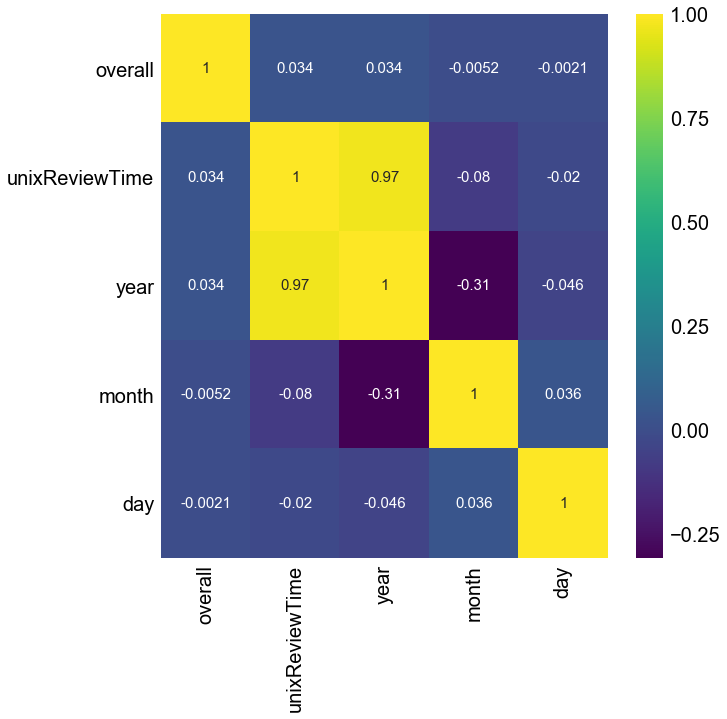#### Memory based Collaborative Filtering¶

Construct the user-to-item matrix

In :
#the shape of a vector is equivalent to
# its length
n_users = df['reviewerID'].unique().shape
n_items = df['asin'].unique().shape

n_ratings = df['overall'].unique().shape

print('Number of users =  {} | Number of items = {}'.format(str(n_users), str(n_items)))

print ('%i Number of unique ratings' %n_ratings)

Number of users =  19856 | Number of items = 8510
5 Number of unique ratings


#### Singular Value Decomposition¶

If a matrix $A$ has a matrix of eigenvectors $P$ that is not invertible then $A$ does not have an eigen decomposition.The singular value decomposition of an $m\times n$ matrix $A$ is the factorization of $A$ into the product of three matrices

$A=UDV^{T}$.

Where the $U$ is an $m \times m$ matrix and an $n \times n$ matrix $V$ both have orthogonal column vectors such that

$UU^{T}=I$

and

$VV^{T}=I$

the matrix $D$ is an $m \times n$ diagonal matrix with non-negative real numbers on the diagonal. The diagonal elements $\sigma_{i}$ of $D$ are singular values of $A$.The singular values of a matrix A are the square roots of the non-zero eigenvalues of the matrix $A^{T}A$, where $A^{T}$ the transpose-conjugate matrix if it has complex coefficients, or the transpose if it has real coefficients.

Common applications of SVD include Principal Component Analysis, computing the pseudoinverse, least squares fitting of data, multivariable control, matrix approximation, and determining the rank, range and null space of a matrix.

In matrix factorization based recommendation systems,interest is in maintaining the same dimensionality.The matrix factorization is done on the user-item ratings matrix. Matrix factorization can be understood as finding 2 matrices whose product is the original matrix. The $U$ matrix represents the feature vectors corresponding to the users in the hidden feature space and the $V$ matrix represents the feature vectors corresponding to the items in the hidden feature space.

The predictions can be made by taking a dot product of $U, S$ and $V^{T}$.

Each item can be represented by a vector $q_{i}$. Similarly each user can be represented by a vector $p_{u}$ such that the dot product of those 2 vectors is the unbiased expected rating

$\text{The prediction or expected rating :} \hspace{5mm} \hat{r}_{ui}= q_{i}^{T}P_{u}$

$q_{i}$ and $p_{u}$ can be found by minimizing the squared error difference between the observed rating and the expected rating of the user-item matrix.

The addition of a penalty term in the minimization equation reduces overfitting the training set and allows the model to generalize to unseen data. This is represented by a regularization parameter $\lambda$ multiplied by the square sum of the magnitudes of user and item vectors.

$min(P_{u},q_{i}) \displaystyle \sum\limits_{r_{ui} \in R_{train} } \left( \hat{r}_{ui}-r_{ui} \right)^{2} +\lambda \left( \Vert q_{i} \Vert^{2} +\|P_{u}\|^{2}\right)$

The residual error between the predicted and actual value can be reduced by adding some characteristics from the dataset to the prediction model.For each user-item $(u,i)$ pair we can extract 3 parameters. $\mu$ which is the average ratings of all items, $b_{i}$ which is the average rating of item $i$ minus $\mu$ and $b_{u}$ which is the average rating given by user $u$ minus $\mu$ which makes the expected rating:

$\text{The prediction or expected rating :}\hat{r}_{ui}= \mu +b_{u} +b_{i}+ q_{i}^{T}P_{u}$

$q_{i}$ and $p_{u}$ can be found by minimizing the squared error difference between the observed rating and the expected rating of the user-item matrix.

$min(P_{u},q_{i},b_{u},b_{i}) \displaystyle \sum\limits_{r_{ui} \in R_{train} } \left( \hat{r}_{ui}-r_{ui} \right)^{2} +\lambda \left( b_{u}^{2} +b_{i}^{2}+\Vert q_{i} \Vert^{2} +\|P_{u}\|^{2}\right)$

The minimization can be performed by a very stochastic gradient descent or alternating least squares.

In :
from surprise import SVD
from surprise import KNNBasic
from surprise import Dataset
from surprise import accuracy
from surprise.model_selection import KFold
from sklearn.model_selection import train_test_split
from surprise import NormalPredictor
from surprise import Dataset
from surprise.model_selection import cross_validate

trainset, testset = train_test_split(df, test_size=0.20)

# A reader is still needed but only the rating_scale param is required.
# The Reader class is used to parse a file containing ratings.

# The columns must correspond to user id, item id and ratings (in that order).

traindata.split(n_folds=5)

from surprise import SVD, evaluate
from surprise import NMF

algo = SVD()

evaluate(algo, traindata, measures=['RMSE', 'MAE'])

#algo = NMF()
#evaluate(algo, traindata, measures=['RMSE', 'MAE'])
#algorithms = (SVD, KNNBasic, KNNWithMeans, NormalPredictor)
#pd.DataFrame.as_matrix(testset)
#df.values

predictions = algo.test(testset[['reviewerID', 'asin', 'overall']].values)

# Then compute RMSE
accuracy.rmse(predictions)
accuracy.mae(predictions)

Evaluating RMSE, MAE of algorithm SVD.

------------
Fold 1
RMSE: 1.1233
MAE:  0.8634
------------
Fold 2
RMSE: 1.1202
MAE:  0.8586
------------
Fold 3
RMSE: 1.1114
MAE:  0.8547
------------
Fold 4
RMSE: 1.1209
MAE:  0.8600
------------
Fold 5
RMSE: 1.1068
MAE:  0.8520
------------
------------
Mean RMSE: 1.1165
Mean MAE : 0.8577
------------
------------
RMSE: 1.1146
MAE:  0.8564

Out:
0.8564350767699659

Non-negative matrix factorization is a linear, non-negative approximate data representation. Let $T$ be measurements of $N$ non-negative scalar variables of some data. For an $N$ dimensional measurement vectors v^{t}\hspace{5mm} t=1,\cdots, T$a linear approximation of the data is given by :$v^{T} \approx \displaystyle \sum\limits_{i=1}^{M}W_{i}h_{i}^{t}=Wh^{t}$In matrix notation$ V \approx WH $where$W$is an$N \times M $matrix with basis vectors$w_{i}$on its columns and where each column of$H$contains the coefficient vector$h^{t}$corresponding to the measurement vector$v^{t}$.All three matrices exhibit the property that they have no negative elements. some common applications of NMF include astronomy, computer vision, document clustering, chemometrics, audio signal processing, recommender systems and bioinformatics. In order to construct recommendations, user$\times$item matrix is constructed such that every element is a rating if it exist. In : from surprise import SVD from surprise import KNNBasic from surprise import Dataset from surprise import accuracy from surprise.model_selection import KFold from sklearn.model_selection import train_test_split from surprise import NormalPredictor from surprise import Dataset from surprise import Reader from surprise.model_selection import cross_validate trainset, testset = train_test_split(df, test_size=0.20) # A reader is still needed but only the rating_scale param is required. # The Reader class is used to parse a file containing ratings. # path to dataset file #file_path = '/home/nico/.surprise_data/ml-100k/ml-100k/u.data' # change this # As we're loading a custom dataset, we need to define a reader. In the # movielens-100k dataset, each line has the following format: # 'user item rating timestamp', separated by '\t' characters. #reader = Reader(line_format='user item rating timestamp', sep='\t') #data = Dataset.load_from_file(file_path, reader=reader) #data.split(n_folds=5) reader = Reader(rating_scale=(1, 5)) # The columns must correspond to user id, item id and ratings (in that order). traindata = Dataset.load_from_df(trainset[['reviewerID', 'asin', 'overall']], reader) traindata.split(n_folds=5) from surprise import SVD, evaluate from surprise import NMF algo = NMF() evaluate(algo, traindata, measures=['RMSE', 'MAE']) #algo = NMF() #evaluate(algo, traindata, measures=['RMSE', 'MAE']) #algorithms = (SVD, KNNBasic, KNNWithMeans, NormalPredictor) #pd.DataFrame.as_matrix(testset) #df.values predictions = algo.test(testset[['reviewerID', 'asin', 'overall']].values) # Then compute RMSE accuracy.rmse(predictions) accuracy.mae(predictions)  Evaluating RMSE, MAE of algorithm NMF. ------------ Fold 1 RMSE: 1.3465 MAE: 1.0438 ------------ Fold 2 RMSE: 1.3346 MAE: 1.0362 ------------ Fold 3 RMSE: 1.3275 MAE: 1.0339 ------------ Fold 4 RMSE: 1.3375 MAE: 1.0402 ------------ Fold 5 RMSE: 1.3333 MAE: 1.0354 ------------ ------------ Mean RMSE: 1.3359 Mean MAE : 1.0379 ------------ ------------ RMSE: 1.3359 MAE: 1.0386  Out: 1.038581461461138 In : pd.DataFrame(predictions).head()  Out: uid iid r_ui est details 0 A3ENX1Y48DJQVK B004ZJOKJE 5.0 3.748853 {'was_impossible': False} 1 A852I21I0KLM0 B00074W45S 4.0 2.025169 {'was_impossible': False} 2 A23X105IJ30KBW B0009YHTA6 4.0 3.841245 {'was_impossible': False} 3 A3LWC833HQIG7J B004EEN5BE 5.0 4.236177 {'was_impossible': False} 4 A28ETU4J2PG09N B0011DHJ2W 4.0 3.823349 {'was_impossible': False} We can obtain the rating prediction for single user as below: In : #uid = str(A2K2BWL8PYPV3S) # raw user id (as in the ratings file). They are **strings**! #iid = str(B0042CVBNM) # raw item id (as in the ratings file). They are **strings**! # get a prediction for specific users and items. l=testset[['reviewerID', 'asin', 'overall']].values uid=l iid=l r=l pred = algo.predict(uid, iid, r, verbose=True )  user: A2K2BWL8PYPV3S item: B0042CVBNM r_ui = 2.00 est = 3.75 {'was_impossible': False}  #### SVD With scipy¶ Create User-Item Matrix In : user_item = df.pivot(index = 'reviewerID', columns ='asin', values = 'overall').fillna(0) user_item_matrix=user_item.as_matrix( ) user_item_matrix[0:4,0:5]  Out: array([[0., 0., 0., 0., 0.], [0., 0., 0., 0., 0.], [0., 0., 0., 0., 0.], [0., 0., 0., 0., 0.]]) #### Matrix Sparsity (Density)¶ The percentage on non-zero elements to the number of of elements in total (1-the ratio of non-zero elements to the number of elemnts).If most of the elements are nonzero, then the matrix is considered dense$\text{sparsity} = \frac{\text{count zero elements}}{ \text{total elements}} =1-\text{density}$In : # several ways to find sparsity of a matrix from numpy import count_nonzero sparsity =(1.0 - count_nonzero(user_item_matrix) / user_item_matrix.size)*100 print('The sparsity of the data is {} %'.format(sparsity)) sparsity=round(1.0-len(df)/float(n_users*n_items),10)*100 print('The sparsity of the data is {} %'.format(sparsity)) sparsity = float(len((user_item_matrix).nonzero())) sparsity /= (user_item_matrix.shape * user_item_matrix.shape) sparsity = 1-sparsity sparsity *= 100 print('The sparsity of the data is {} %'.format(sparsity)) sparsity = float(len((user_item_matrix).nonzero())) sparsity /= (user_item_matrix.shape * user_item_matrix.shape) sparsity *= 100 print ('percentage of user-items that have a rating: {:.5f}%'.format(sparsity))  The sparsity of the data is 99.90659185619421 % The sparsity of the data is 99.90659186 % The sparsity of the data is 99.90659185619421 % percentage of user-items that have a rating: 0.09341%  In :  # convert to sparse matrix (CSR method) from scipy.sparse import csr_matrix user_item_sparse=csr_matrix(user_item_matrix) # reconstruct dense matrix #user_item_sparse.todense()  #### Split user_item_matrix into train and test set¶ In : trainset_data, testset_data = train_test_split(user_item_sparse, test_size=0.20)  In : #A=trainset_user.as_matrix() A=trainset_data user_ratings_mean = np.mean(A, axis = 1) A_demeaned = A - user_ratings_mean.reshape(-1, 1)  In : from scipy.sparse.linalg import svds U, D, Vt = svds(A_demeaned, k = 50) D = np.diag(D)  In : user_predicted_ratings = np.dot(np.dot(U, D), Vt) + user_ratings_mean.reshape(-1, 1) user_predicted_ratings[0:2,0:5]  Out: matrix([[ 0.00259524, 0.00248465, 0.00044646, -0.00311194, 0.00474133], [ 0.0044355 , 0.00497795, 0.00702899, 0.00386226, 0.0045359 ]]) In : prediction = pd.DataFrame(user_predicted_ratings, columns = user_item.columns )  In : prediction.iloc[0:4,0:8]  Out: asin 1223000893 4847676011 9376674824 B00002N8FK B00004X14K B0000537O8 B00005MF9T B00005MF9U 0 0.000245 0.000134 -0.001904 -0.005462 0.002391 -0.001560 -0.000020 0.000344 1 0.001380 0.001923 0.003974 0.000807 0.001481 0.000725 0.002222 -0.000107 2 0.000193 0.001999 -0.001699 0.001071 0.001679 0.000057 0.006172 0.000044 3 0.002832 0.003529 0.002921 0.003183 0.003188 0.003830 0.004979 0.003130 In : from sklearn.metrics import mean_squared_error from math import sqrt def rmse(prediction, ground_truth): prediction = prediction[ground_truth.nonzero()].flatten() ground_truth = ground_truth[ground_truth.nonzero()].flatten() return sqrt(mean_squared_error(prediction, ground_truth))  The pairwise_distances function from sklearn can calculate the cosine similarity. The output ranges from 0 to 1 since the ratings are all positive. Memory-Based Collaborative Filtering This creats a validation dataset by selecting rows (user) that have 35 or more ratings, then randomly select 15 of those ratings for validation set, but set those values to 0 in the training set. In : def train_test_splitt(user_item_matrix): validation = np.zeros(user_item_matrix.shape) train = user_item_matrix.copy() for user in np.arange(user_item_matrix.shape): # 35 seems to be best, it depends on sparsity of your user-item matrix if len(user_item_matrix[user,:].nonzero())>=35: val_ratings = np.random.choice(user_item_matrix[user, :].nonzero(), size=15, #tweak this, 15 seems to be optimal replace=False) train[user, val_ratings] = 0 validation[user, val_ratings] = user_item_matrix[user, val_ratings] print(validation.shape) return train, validation  In : train, val = train_test_split(user_item_matrix)  In : from numpy import linalg as LA  In : def cos_similarity(user_item_matrix, kind='user', epsilon=1e-9): # epsilon -> small number for handling division-by-zero errors if kind == 'user': sim = user_item_matrix.dot(user_item_matrix.T) + epsilon norms=np.array(LA.norm(user_item_matrix)*LA.norm(user_item_matrix.T)) elif kind == 'item': sim = user_item_matrix.T.dot(user_item_matrix) + epsilon norms = np.array(LA.norm(user_item_matrix.T)*LA.norm(user_item_matrix)) return (sim / norms )  In : user_similarity = cos_similarity(train, kind='user') item_similarity=cos_similarity(train, kind='item')  In : user_similarity[0:4,0:5]  Out: array([[4.60462497e-05, 4.38535712e-16, 4.38535712e-16, 4.38535712e-16, 4.38535712e-16], [4.38535712e-16, 4.38535712e-05, 4.38535712e-16, 4.38535712e-16, 4.38535712e-16], [4.38535712e-16, 4.38535712e-16, 8.37603209e-05, 4.38535712e-16, 4.38535712e-16], [4.38535712e-16, 4.38535712e-16, 4.38535712e-16, 5.17472140e-05, 4.38535712e-16]]) In : def predict_nobias(user_item_matrix, similarity, kind='user'): if kind == 'user': user_bias = user_item_matrix.mean(axis=1) user_item_matrix = (user_item_matrix - user_bias[:, np.newaxis]).copy() pred = similarity.dot(user_item_matrix) / np.array([np.abs(similarity).sum(axis=1)]).T pred += user_bias[:, np.newaxis] elif kind == 'item': item_bias = user_item_matrix.mean(axis=0) user_item_matrix = (user_item_matrix - item_bias[np.newaxis, :]).copy() pred = user_item_matrix.dot(similarity) / np.array([np.abs(similarity).sum(axis=1)]) pred += item_bias[np.newaxis, :] return pred  In : user_prediction2 = predict_nobias(train, user_similarity, kind='user') item_prediction2 = predict_nobias(train, item_similarity, kind='item')  In : user_prediction2[0:3,0:5]  Out: array([[-0.00346554, -0.00346554, 0.01601102, -0.00346554, -0.00346554], [-0.00184352, -0.00184352, -0.00184352, -0.00184352, -0.00184352], [-0.00328262, -0.00328262, -0.00328262, 0.01465996, -0.00328262]]) In : from sklearn.metrics import mean_squared_error from math import sqrt def rmse(prediction, ground_truth): prediction = prediction[ground_truth.nonzero()].flatten() ground_truth = ground_truth[ground_truth.nonzero()].flatten() return sqrt(mean_squared_error(prediction, ground_truth))  In : print ('User-based bias-adjusted CF RMSE: %.2f' %rmse(user_prediction2, val)) print ('Item-based bias-adjusted CF RMSE: %.2f' %rmse(item_prediction2, val))  User-based bias-adjusted CF RMSE: 4.37 Item-based bias-adjusted CF RMSE: 4.38  #### Model based collaborative filtering - matrix factorization (via SVD)¶ In : import scipy.sparse as sp from scipy.sparse.linalg import svds  In : #get SVD components from train matrix. Choose k. u, s, vt = svds(train, k = 50)# dimensionality for rank matrix s_diag_matrix=np.diag(s) X_pred = np.dot(np.dot(u, s_diag_matrix), vt)  In : print ('matrix-factorization CF RMSE: %.2f' %rmse(X_pred, val))  matrix-factorization CF RMSE: 4.38  In : pd.DataFrame(X_pred, columns = user_item.columns).iloc[0:4,0:9]  Out: asin 1223000893 4847676011 9376674824 B00002N8FK B00004X14K B0000537O8 B00005MF9T B00005MF9U B00005MF9V 0 -0.000175 0.000376 0.000690 0.000055 0.000143 -0.000173 -0.000036 -0.000591 -0.000131 1 -0.000057 0.000029 0.000178 0.000055 0.000020 0.000152 0.000159 0.000077 0.000037 2 -0.000085 -0.000089 0.000494 0.000172 0.000142 -0.000291 -0.000083 -0.000241 -0.000128 3 -0.000268 0.002196 0.009465 0.000147 0.000195 -0.002036 -0.000545 0.000361 0.000305 #### Using Stochastic Gradient Descent method to update the above low rank matrices for the latent features (online learning).¶ Stochastic gradient descent (SGD), also known as incremental gradient descent, is an iterative method for optimizing a differentiable objective function, a stochastic approximation of gradient descent optimization. The standard gradient descent algorithm updates the parameters θ of the objective$J(θ)$as,$\theta=\theta-\eta \nabla_{\theta}E[J(\theta)] $where${\displaystyle \eta } $is a step size (learning rate in machine learning). The expectation in the above equation is approximated by evaluating the cost and gradient over the full training set. Stochastic Gradient Descent (SGD) replaces the expectation in the update and computes the gradient of the parameters using only a single or a few randomly shuffled examples in the training set. The new update is given by,$\theta=\theta-\eta \nabla_{\theta}J(\theta;x^{(i)},y^{(i)}) $with a pair$ (x(i),y(i))$from the training set. In : #P is latent user feature matrix #Q is latent item feature matrix def prediction(P,Q): return np.dot(P.T,Q)  In : lmbda = 0.4 # Regularization parameter k = 3 #tweak this parameter m, n = train.shape # Number of users and items n_epochs = 100 # Number of epochs eta=0.01 # Learning rate P = 3 * np.random.rand(k,m) # Latent user feature matrix Q = 3 * np.random.rand(k,n) # Latent movie feature matrix  In : train_errors = [] val_errors = [] #Only consider items with ratings users,items = train.nonzero() for epoch in range(n_epochs): for u, i in zip(users,items): e = train[u, i] - prediction(P[:,u],Q[:,i]) # Calculate error for gradient update P[:,u] += eta * ( e * Q[:,i] - lmbda * P[:,u]) # Update latent user feature matrix Q[:,i] += eta * ( e * P[:,u] - lmbda * Q[:,i]) # Update latent item feature matrix train_rmse = rmse(prediction(P,Q),train) val_rmse = rmse(prediction(P,Q),val) train_errors.append(train_rmse) val_errors.append(val_rmse)  In : import matplotlib.pyplot as plt %matplotlib inline sns.set(rc={"font.style":"normal", #"axes.facecolor":bg_color, "axes.titlesize":30, #"figure.facecolor":bg_color, "text.color":"black", "xtick.color":"black", "ytick.color":"black", "axes.labelcolor":"black", "axes.grid":True, 'axes.labelsize':20, 'figure.figsize':(10.0, 7.0), 'xtick.labelsize':25, 'legend.fontsize': 16, 'legend.handlelength': 2, 'ytick.labelsize':20}) plt.plot(range(n_epochs), train_errors, marker='o', label='Training Data'); plt.plot(range(n_epochs), val_errors, marker='v', label='Validation Data'); plt.xlabel('Number of Epochs'); plt.ylabel('RMSE'); plt.legend() plt.grid() plt.show()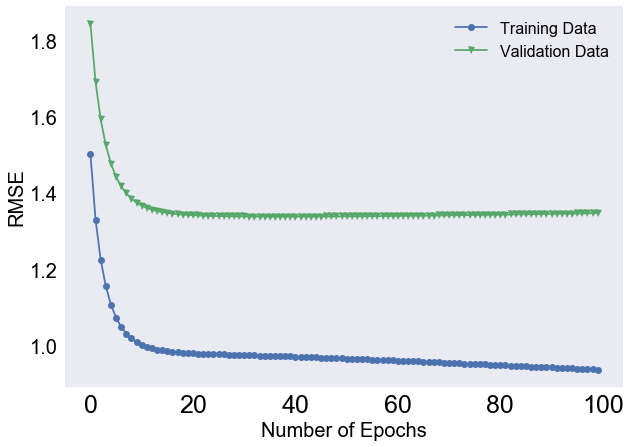#### Take a look at prediction vs. actual ratings¶ In : SGD_prediction=prediction(P,Q)  In : estimation= SGD_prediction[val.nonzero()] ground_truth = val[val.nonzero()] results=pd.DataFrame({'prediction':estimation, 'actual rating':ground_truth})  In : results.head()  Out: actual rating prediction 0 5.0 3.131683 1 5.0 3.238381 2 5.0 2.627644 3 5.0 2.944819 4 1.0 3.115936 #### Finding Optimal k (dimensionality rank for matrix)¶ #### Top-k Collaborative Filtering¶ The prediction MSE can be improved by considering the top k users who are most similar to the input user (or, similarly, the top$k$items). That is, when we calculate the sums over$u′\$

\begin{equation*} \hat{r}_{u,i}= \frac{\displaystyle \sum\limits_{u^{\prime} \in U }\text{Similarity }(u,u^{\prime})r_{u^{\prime},i}}{\displaystyle \sum\limits_{u^{\prime} \in U }\left|\text{Similarity }(u,u^{\prime})\right|} \end{equation*}

The sum is over the top k most similar users. Parameter tuning can be used to find the optimal value for minimizing RMSE in the test set.

In :
def predict_topk(user_item_matrix, similarity, kind='user', k=40):
pred = np.zeros(user_item_matrix.shape)
if kind == 'user':
for i in range(user_item_matrix.shape):
top_k_users = [np.argsort(similarity[:,i])[:-k-1:-1]]
for j in range(user_item_matrix.shape):
pred[i, j] = similarity[i, :][top_k_users].dot(user_item_matrix[:, j][top_k_users])
pred[i, j] /= np.sum(np.abs(similarity[i, :][top_k_users]))
if kind == 'item':
for j in range(user_item_matrix.shape):
top_k_items = [np.argsort(similarity[:,j])[:-k-1:-1]]
for i in range(user_item_matrix.shape):
pred[i, j] = similarity[j, :][top_k_items].dot(user_item_matrix[i, :][top_k_items].T)
pred[i, j] /= np.sum(np.abs(similarity[j, :][top_k_items]))

return pred

In :
from sklearn.metrics import mean_squared_error

def get_rmse(pred, actual):
# Ignore nonzero terms.
pred = pred[actual.nonzero()].flatten()
actual = actual[actual.nonzero()].flatten()
return sqrt(mean_squared_error(pred, actual))

In :
pred = predict_topk(train, user_similarity, kind='user', k=40)
print('Top-k User-based CF MSE: {}'.format(str(get_rmse(pred, val))))

pred = predict_topk(train, item_similarity, kind='item', k=40)

print('Top-k Item-based CF MSE: {}'.format(str(get_rmse(pred, val))))

Top-k User-based CF MSE: 4.3683132619552465
Top-k Item-based CF MSE: 4.370556179982666

In :
k_array = [5, 15, 30, 50, 100, 200]
user_train_rmse = []
user_test_rmse = []
item_test_rmse = []
item_train_rmse = []

for k in k_array:
user_pred = predict_topk(train, user_similarity, kind='user', k=k)
item_pred = predict_topk(train, item_similarity, kind='item', k=k)

user_train_rmse += [get_rmse(user_pred, train)]
user_test_rmse += [get_rmse(user_pred, val)]

item_train_rmse += [get_rmse(item_pred, train)]
item_test_rmse += [get_rmse(item_pred, val)]

In :
%matplotlib inline
import matplotlib.pyplot as plt
import seaborn as sns
sns.set()

pal = sns.color_palette("viridis", 2)

plt.figure(figsize=(12, 8))

plt.plot(k_array, user_train_rmse, c=pal, label='User-based train', alpha=0.5, linewidth=5)
plt.plot(k_array, user_test_rmse, c=pal, label='User-based test', linewidth=5)
plt.plot(k_array, item_train_rmse, c=pal, label='Item-based train', alpha=0.5, linewidth=5)
plt.plot(k_array, item_test_rmse, c=pal, label='Item-based test', linewidth=5)
plt.legend(loc='best', fontsize=20)
plt.xticks(fontsize=16);
plt.yticks(fontsize=16);
plt.xlabel('K', fontsize=20);
plt.ylabel('RMSE', fontsize=20);